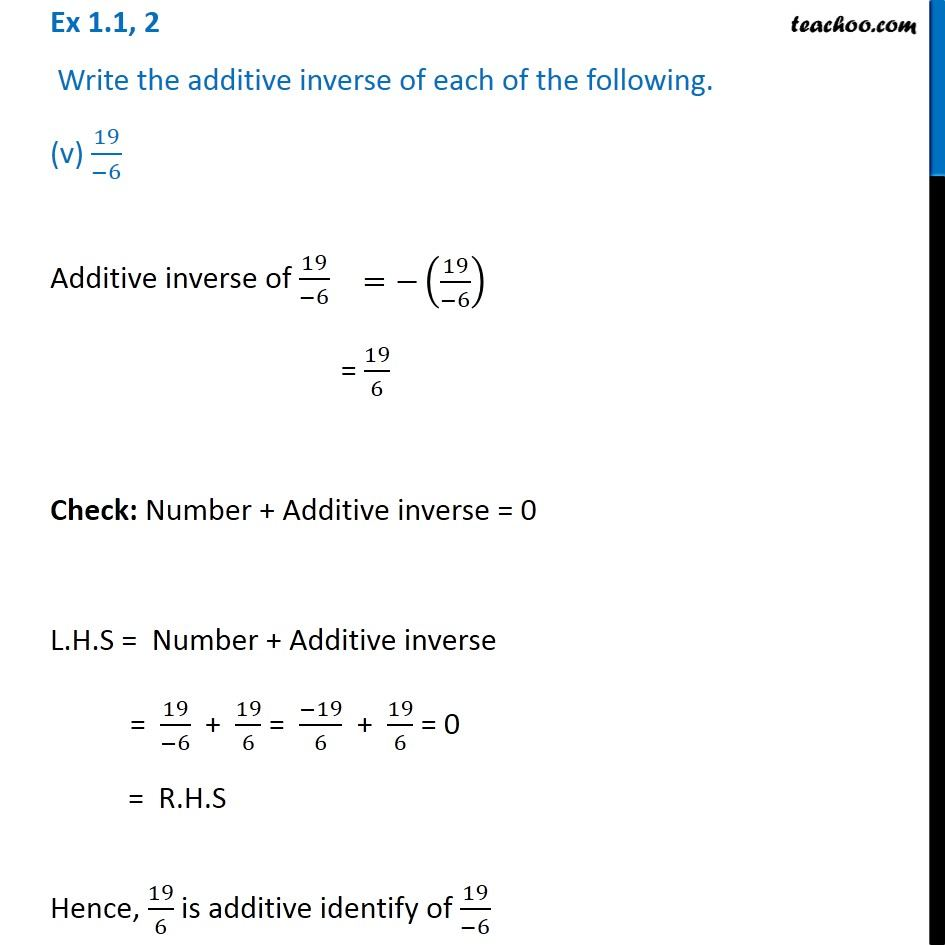Ex 1.1

Chapter 1 Class 8 Rational Numbers
Serial order wiseLearn in your speed, with individual attention - Teachoo Maths 1-on-1 Class

### Transcript

Ex 1.1, 2 Write the additive inverse of each of the following. (v) 19/( 6) Additive inverse of 19/( 6) = 19/6 Check: Number + Additive inverse = 0 L.H.S = Number + Additive inverse = 19/( 6) + 19/6 = ( 19)/6 + 19/6 = 0 = R.H.S Hence, 19/6 is additive identify of 19/( 6)